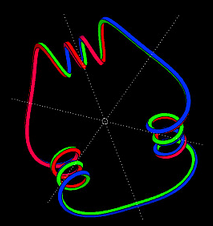# Constant Torsion Curve

Back to interactive Constant Torsion CurvesConstant torsion morph Geometric invariants of curves are easier to explain when the curve is parametrized by arc length. Then the torsion function of the space curve measures, how fast the second derivative of the curve rotates around the curve. The animation shows curves of constant torsion for which the curvature is a trigonometric polynomial. For certain coefficients of the polynomial these curves are closed curves - there are three of them in this animation.

Whether the curve closes or not, we know from the curvature function for which parameter values 180 degree rotation about the principal normal of the curve is a symmetry. The construction of closed curves proceeds in two steps: first we choose parameters so that two neighboring symmetry normals intersect. Then all symmetry normals pass through this point. Then the parameters are further restricted so that the angle between neighboring symmetry normals is a rational number times pi, for 3-fold symmetry we adjust to pi/3, for 4-fold symmetry we adjust to pi/4.